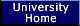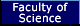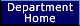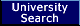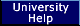## Department of Computer Science# GAP3 Program for Computing Left Kan Extensions

 Contents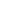The Computerised Rewriting Procedure for Kan ExtensionsExampleExplanationContact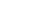Links to other pagesComputational Category TheoryCategorical Rewriting GroupSearch## The Computerised Rewriting Procedure for Kan Extensions

Let A and B be categories, and let F:A->B be a functor. An action of the category is a functor X:A->Sets. The extension of this action X of A along F to B, satisfying the universal property is called the Kan extension of X along F.

Combinatorially, categories can be presented by generating graphs and sets of relations on the paths in the graphs, and functors can be defined on the generating graphs of the source categories. Combinatorial data presenting a Kan extension consists of a generating graph for A, a generating graph for B together with a set of relations on its paths, X is defined by allocating one set to each object of A's graph and a function for each arrow (between the appropriate sets), finally F is defined by allocating an object of B for each object of A and a path of B for each arrow of A. In the computer program this data is given by a record containing 9 fields. The fields are as follows (all lists are ordered lists, corresponding is used to mean elements are in the same position):

ObA: A list of integers for the objects of A.

ArrA: A list of pairs of objects of A, one [source,target] pair for each arrow.

ObB: A list of integers for the objects of B.

ArrB: A list of triples, one [label,source,target] triple for each arrow of B, the labels must be distinct variables and the other entries are integers representing objects of B.

RelB: A list of relations, one [path,path] pair for each relation of B.

FObA: A list with the same number of entries as ObA, the entry corresponding to the object A1 is the image of A1 under F.

FArrA: A list with the same number of entries as ArrA, the entry corresponding to the arrow a1 is the image of a1 under F.

XObA: A list with the same number of entries as ObA, the entry corresponding to A1 is a list of distinct variables representing the set that is the image of A1 under X.

XArrA:=A list with the same number of entries as ArrA, the entry corresponding to the arrow a1 is a list whose entries are the images under the function X(a1) of the set X(src(a1)).

In the paper ``Using Rewrite Systems to Compute Kan Extensions of Actions of Categories'' by R. Brown, A.Heyworth and L. Lambe, we define a set T and a rewrite system R such that if R is completed the Kan extension can be computed.

Let P be the free category on the generating graph of B. The the set T which consists of all possible terms x|b1*...*bn so that b1*...*bn is a path in P and x is an element of a set which is the image under X of an object of A whose image under F is the source of b1. The free category P acts on the right of the elements of T by composition. Let RP be the set of relations of B and let RT be the set of relations (x|Fa1,Xa1(x)|id) where a1 is an arrow of A, x is an element of X(src(a1)) and id is the identity arrow at tgt(Fa1). The union of RR and RT defines a rewrite system on T. If T is factored by the congruence generated by this rewrite system then the congruence classes, with the action of B, defines the Kan extension. This is only a vague outline of the ideas which are explained clearly, in detail, with some interesting examples, in the paper.

## Example

The following is a small example of a GAP3 session to attempt to compute a Kan extension of an action. Here A has two objects (1,2) and two arrows ([1,2],[2,1]); B has three objects (1,2,3) and five (labelled) arrows ([b1,1,2],[b2,2,3],[b3,3,1],[b4,1,1],[b5,1,3]) and one relation (b1*b2*b3=b4); X maps the first object of A to the set {x1,x2,x3} and the second to {y1, y2}, the first arrow of A gives (under X) the function x1 -> y1, x2 -> y2, x3 ->y1, and the second gives the function y1 -> x1, y2 -> x2; Finally F maps the first and second objects of A to the first and second objects of B respectively, and the first arrow of A is mapped by F to b1 and the second to b2*b3.

The input to the computer program takes the following form. First we set up the variables:

gap> F:=FreeGroup("b1","b2","b3","b4","b5","x1","x2","x3","y1","y2");;

gap> b1:=F.1;;b2:=F.2;;b3:=F.3;;b4:=F.4;;b5:=F.5;; x1:=F.6;;x2:=F.7;;x3:=F.8;;y1:=F.9;;y2:=F.10;;

Then we input the data:

gap> ObA:=[1,2];;

gap> ArrA:=[[1,2],[2,1]];;

gap> ObB:=[1,2,3];;

gap> ArrB:=[[b1,1,2],[b2,2,3],[b3,3,1],[b4,1,1],[b5,1,3]];;

gap> RelB:=[[b1*b2*b3,b4]];;

gap> FObA:=[1,2];;

gap> FArrA:=[b1,b2*b3];;

gap> XObA:=[[x1,x2,x3],[y1,y2]];;

gap> XArrA:=[[y1,y2,y1],[x1,x2]];;

To combine all this data in one record do:

gap> KAN:=rec( ObA:=ObA, ArrA:=ArrA, ObB:=ObB, ArrB:=ArrB, RelB:=RelB, FObA:=FObA, FArrA:=FArrA, XObA:=XObA, XArrA:=XArrA );;

To calculate the initial rules do

gap> InitialRules( KAN );

The output will be

[ [ b1*b2*b3, b4 ], [ x1*b1, y1 ], [ x2*b1, y2 ], [ x3*b1, y1 ], [ y1*b2*b3, x1 ], [ y2*b2*b3, x2 ] ]

This means that there are five initial \$\ep\$-rules and one initial \$K\$-rule. To attempt to complete the Kan extension presentation do:

gap> KB( InitialRules(KAN) );

The output is:

[ [ x1*b1, y1 ], [ x1*b4, x1 ], [ x2*b1, y2 ], [ x2*b4, x2 ], [ x3*b1, y1 ], [ x3*b4, x1 ], [ b1*b2*b3, b4 ], [ y1*b2*b3, x1 ], [ y2*b2*b3, x2 ] ]

This is a complete rewrite system

The result of attempting to compute the sets (`the sets' being the images Ki of the objects i under the new action K:B->Sets that is the Kan extension) by typing:

gap> Kan(KAN);

is a long list and then:

enumeration limit exceeded: complete rewrite system is:

[ [ x1*b1, y1 ], [ x1*b4, x1 ], [ x2*b1, y2 ], [ x2*b4, x2 ], [ x3*b1, y1 ], [ x3*b4, x1 ], [ b1*b2*b3, b4 ], [ y1*b2*b3, x1 ], [ y2*b2*b3, x2 ] ]

This means that the sets Ki for i in B are too large (the limit set in the program is 1000, which can be changed by typing EnumerationLimit:=5000; (or whatever) after reading the program). In fact this example isinfinite. The complete rewrite system is output instead of the sets. We can in fact use this to obtain a regular expression for each of the sets. In this case the regular expressions are:

K1=(x1+x2+x3)|(b5(b3{b4}^*b5)^*b3{b4}^*+id,

K2=(x1+x2+x3)|b5(b3{b4}^*b5)^*b3{b4}^*(b1) +(y1+y2)|id,

K3=(x1+x_2+x_3)|b5(b3{b4}^*b5)^*(b3{b4}^*b1b2+id) + (y1 + y2)|b2.

The actions of the arrows are defined by concatenation followed by reduction. For example x1|b5*b3*b4*b4*b5 is an element of K3, so b3 acts on it to give x1|b5*b3*b4*b4*b5*b3 which is irreducible, and an element of K1.

## Explanation

You can find a more detailed explanation of this work here.

## Contact